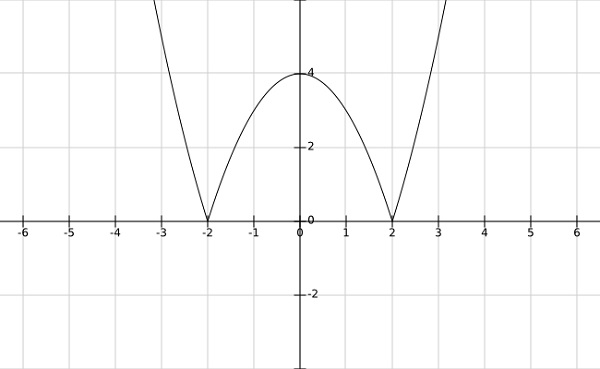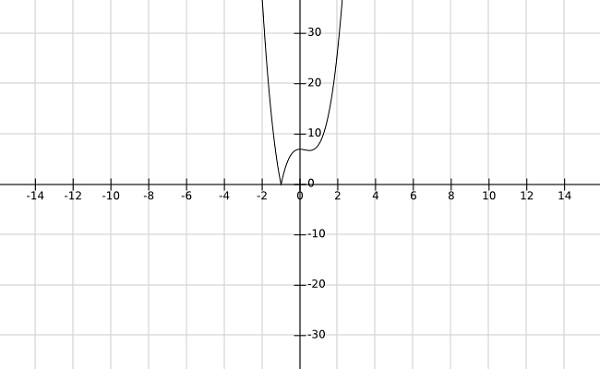# Convex Optimization - Minima and Maxima

## Local Minima or Minimize

$\bar{x}\in \:S$ is said to be local minima of a function $f$ if $f\left ( \bar{x} \right )\leq f\left ( x \right ),\forall x \in N_\varepsilon \left ( \bar{x} \right )$ where $N_\varepsilon \left ( \bar{x} \right )$ means neighbourhood of $\bar{x}$, i.e., $N_\varepsilon \left ( \bar{x} \right )$ means $\left \| x-\bar{x} \right \|< \varepsilon$

## Local Maxima or Maximizer

$\bar{x}\in \:S$ is said to be local maxima of a function $f$ if $f\left ( \bar{x} \right )\geq f\left ( x \right ), \forall x \in N_\varepsilon \left ( \bar{x} \right )$ where $N_\varepsilon \left ( \bar{x} \right )$ means neighbourhood of $\bar{x}$, i.e., $N_\varepsilon \left ( \bar{x} \right )$ means $\left \| x-\bar{x} \right \|< \varepsilon$

## Global minima

$\bar{x}\in \:S$ is said to be global minima of a function $f$ if $f\left ( \bar{x} \right )\leq f\left ( x \right ), \forall x \in S$

## Global maxima

$\bar{x}\in \:S$ is said to be global maxima of a function $f$ if $f\left ( \bar{x} \right )\geq f\left ( x \right ), \forall x \in S$

### Examples

Step 1 − find the local minima and maxima of $f\left ( \bar{x} \right )=\left | x^2-4 \right |$

SolutionFrom the graph of the above function, it is clear that the local minima occurs at $x= \pm 2$ and local maxima at $x = 0$

Step 2 − find the global minima af the function $f\left (x \right )=\left | 4x^3-3x^2+7 \right |$

SolutionFrom the graph of the above function, it is clear that the global minima occurs at $x=-1$.